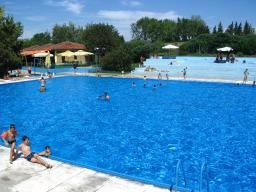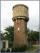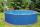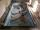# Swimming pool

The pool shape of a cuboid is 299 m3 full of water. Determine the dimensions of its bottom if the water depth is 282 cm and one bottom dimension is 4.7 m greater than the second.

a =  8.21 m
b =  12.91 m

### Step-by-step explanation:Did you find an error or inaccuracy? Feel free to write us. Thank you!Tips to related online calculators
Looking for help with calculating roots of a quadratic equation?
Tip: Our volume units converter will help you with the conversion of volume units.

## Related math problems and questions:

• The poolThe cube-shaped pool has 140 cubic meters of water. Determine the dimensions of the bottom if the depth of the water is 200 cm and one dimension of the base is 3 m greater than the other. What are the dimensions of the pool bottom?
• Swimming poolThe swimming pool has the shape of a block with dimensions of 70dm, 25m, 200cm. How many hl of water can fit into the pool?
• The cuboidThe cuboid is full to the brim with water. The external dimensions are 95 cm, 120 cm, and 60 cm. The thickness of all walls and the bottom is 5 cm. How many liters of water fit into the cuboid?
• Swimming pool 4The pool shaped cuboid measuring 12.5 m × 640 cm at the bottom is 960hl water. To what height in meters reaches the water level?
• Water reservoirThe cuboid reservoir contains 1900 hectoliters of water and the water height is 2.5 m. Determine the dimensions of the bottom where one dimension is 3.2 m longer than the second one.
• Water tankA 288 hectoliter of water was poured into the tank with dimensions 12 m and 6 m bottom and 2 m depth. What part of the volume of the tank water occupied? Calculate the surface of tank wetted with water.
• Cuboid wallsCalculate the cuboid volume if its different walls have an area of 195cm², 135cm², and 117cm².
• Water tankWhat is the height of the cuboid-shaped tank with the bottom dimensions of 80 cm and 50 cm if the 480 liters of water reach 10 cm below the top?
• The rainwaterThe rainwater container has the shape of a block whose bottom has dimensions of 4.5 m and 3.5 m. It is partly filled with water. What is the level if there is 189 hl of water in it?
• Third dimensionCalculate the third dimension of the cuboid: a) V = 224 m3, a = 7 m, b = 4 m b) V = 216 dm3, a = 9 dm, c = 4 dm
• Circular poolThe 3.6-meter pool has a depth of 90 cm. How many liters of water is in the pool?
• Garden poolMr. Novak fill garden pool (cylinder; diameter of 200 cm) with 31.4 hl of water. What is the depth of the pool when the water level is 10 cm below the upper edge of the pool?
• Pool coatingHow many tiles 25 cm × 15 cm need to coat the bottom and sidewalls of the pool with bottom dimensions 30 m × 5 m, if the pool can fit up to 271500 liters of water?
• IcerinkRectangular rink with dimensions of 68.7 m and 561 dm must be covered with a layer of ice 4.2 cm thick. How many liters of water is necessary for the formation of ice when the volume of ice is 9.7% greater than the volume of water.
• PoolThe swimming pool is 10 m wide and 8 m long, and 153 cm deep. How many hectoliters of water are in it if the water is 30 cm below its upper edge?
• TrayWhat height reach water level in the tray shaped a cuboid, if it is 420 liters of water and bottom dimensions are 120 cm and 70 cm.
• Rotary cylinderIn the rotary cylinder it is given: surface S = 96 cm2 and volume V = 192 cm cubic. Calculate its radius and height.